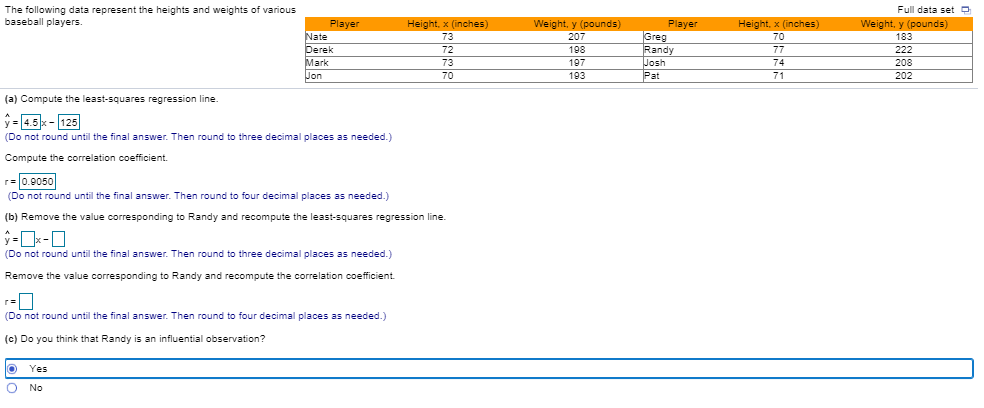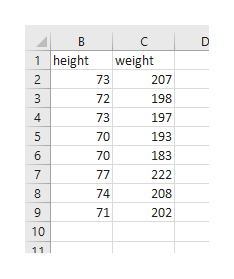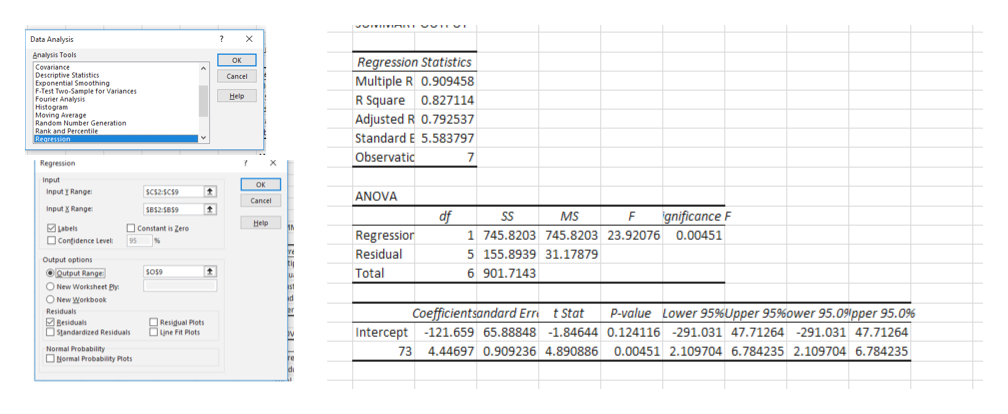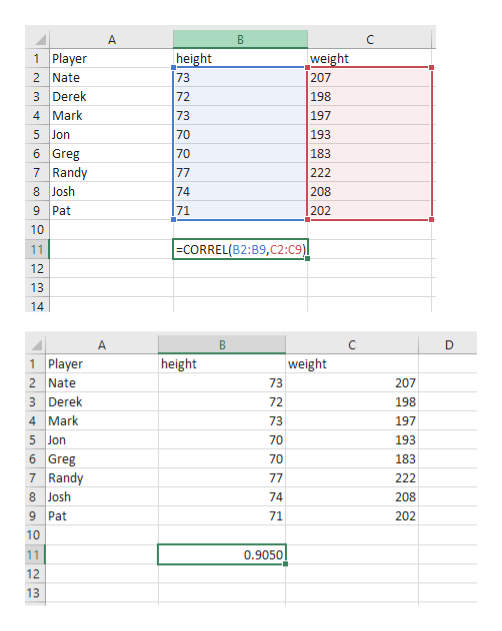Full data setThe following data represent the heights and weights of variousbaseball playersHeight, x (inches)PlayerNateDerekMarkWeight, y (pounds)PlayerGregRandyJoshPatHeight, x (inches)Weight, y (pounds)73207701837273701982227719774208Jon19371202(a) Compute the least-squares regression line.y 4.5x-125(Do not round until the final answer. Then round to three decimal places as needed.)Compute the correlation coefficient.r0.9050(Do not round until the final answer. Then round to four decimal places as needed.)(b) Remove the value corresponding to Randy and recompute the least-squares regression line.-(Do not round until the final answer. Then round to three decimal places as needed.)Remove the value corresponding to Randy and recompute the correlation coefficient.r=(Do not round until the final answer. Then round to four decimal places as needed.)(c) Do you think that Randy is an influential observation?YesO No

Questionhelp_outlineImage TranscriptioncloseFull data set The following data represent the heights and weights of various baseball players Height, x (inches) Player Nate Derek Mark Weight, y (pounds) Player Greg Randy Josh Pat Height, x (inches) Weight, y (pounds) 73 207 70 183 72 73 70 198 222 77 197 74 208 Jon 193 71 202 (a) Compute the least-squares regression line. y 4.5x- 125 (Do not round until the final answer. Then round to three decimal places as needed.) Compute the correlation coefficient. r0.9050 (Do not round until the final answer. Then round to four decimal places as needed.) (b) Remove the value corresponding to Randy and recompute the least-squares regression line. - (Do not round until the final answer. Then round to three decimal places as needed.) Remove the value corresponding to Randy and recompute the correlation coefficient. r= (Do not round until the final answer. Then round to four decimal places as needed.) (c) Do you think that Randy is an influential observation? Yes O No fullscreen
Step 1

Populate excel cells as shown below.help_outlineImage TranscriptioncloseB D 1 height weight 2 73 207 3 72 198 4 73 197 5 70 193 6 70 183 77 7 222 74 208 71 202 10 11 fullscreen
Step 2

Go to Data >Data Analysis> Regression

Fill in input output range.

y= 4.447x -121.659help_outlineImage TranscriptioncloseData Analysis ? X nalysis Tools OK Regression Statistics Multiple R 0.909458 R Square 0.827114 Adjusted R 0.792537 Covariance Descriptive Statistics Exponential Smoothing F-Test Two-Sample for Variances Fourier Analysis Histogram Moving Average Randon Number Generation Rank and Percentile Regression Cancel Help Standard E 5.583797 Observatic 7 Regression Input OK Input y Range SC52SCS ANOVA Cancel Input X Range SB525859 df Regression MS SS gnificance F Help Labels Constant is Zere 1 745.8203 745.8203 23.92076 0.00451 Contidence Level % 95 Residual 5 155.8939 31.17879 Output options Total 6 901.7143 ODutput Range sos9 u O New Worksheet Py ad O New Workbook Residuals Coefficientsandard Err P-value Lower 95%Upper 95%ower 95.09pper 95.0% t Stat Besiduals Standardized Residuals | Resigual Plots une Fit Plots Intercept -121.659 65.88848 -1.84644 0.124116 -291.031 47.71264 -291.031 47.71264 Normal Probability Hormal Probabiity Plots 73 4.44697 0.909236 4.890886 0.00451 2.109704 6.784235 2.109704 6.784235 fullscreen
Step 3

Correlation coefficient can be obtained using C...help_outlineImage TranscriptioncloseA B C 1 Player height 73 weight 207 198 2 Nate 3 Derek 72 4 Mark 73 197 5 Jon 70 193 6 Greg 7 Randy 70 183 77 222 8 Josh 74 208 9 Pat 71 202 10 CORREL(B2:B9,C2:C9)| 11 12 13 14 A 1 Player 2 Nate 3 Derek 4 Mark height weight 73 207 72 198 73 197 5 Jon 70 193 6 Greg 7 Randy 8 Josh 70 183 77 222 74 208 9 Pat 71 202 10 0.9050 11 12 13 fullscreen

Want to see the full answer?

See Solution

Want to see this answer and more?

Our solutions are written by experts, many with advanced degrees, and available 24/7

See Solution
Tagged in

Data